# Piling Up Systems of Linear Equations

## Contextual Tasks for Practicing Solving Systems of Linear Equations

There has been a lot of requests for more contextual and/or 3 act math tasks related to solving systems of linear equations when I work with teachers. A big realization I’ve come to this past year is that making students curious with contextual tasks doesn’t necessarily have to involve a relevant question. Dan Meyer has discussed it multiple times (like here and here), but it still takes a lot of reflection to start identifying what is important when trying to hook your students in.

Continuing the series of Tech Weight and the Tech Weight Sequel, we take the same context of weighing random items to give us some additional practice. You’ll quickly notice that these tasks are definitely contrived, but I still find tasks like these useful if introduced as a challenge to my students. Even though they REALLY don’t care how much each item weighs, they DO enjoy the challenge of solving the problem. The media and 3 act format gives them an easy entry into the task. After allowing students to intuitively solve simple systems of linear equations like in the Counting Candy Sequel or the Tech Weight Sequel, you can use these tasks as a way to practice substitution and/or elimination as a way to solve algebraically.

## Act 1 – Introduce the Task

Show students this video.

Ask students what they are wondering? What questions come to mind?

After discussing with a group, help direct the focus to the question:

How much does each weigh?

Students can make predictions and argue why they believe their guess is best.

## Act 2 – Reveal Some Information

Show students scene 1.

Alternatively, you can show students the following images:

### The Weight of 4 bottles of glue and 5 glue sticks

The weight of 4 bottles of glue and 5 glue sticks is 679 grams.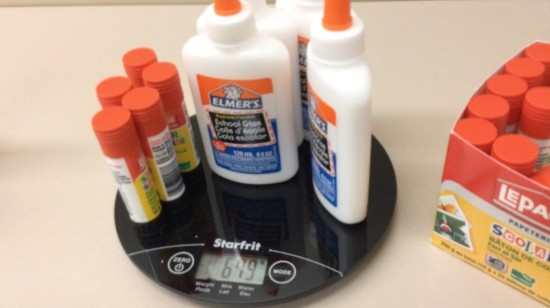### The Weight of 3 bottles of glue and 12 glue sticks

The Weight of 3 bottles of glue and 12 glue sticks is 680 grams.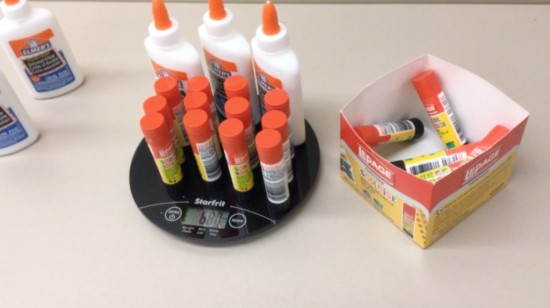Now, students can try to solve this system intuitively, by graphing or algebraically with substitution or elimination.

## Act 3 – Reveal Solution

Show students the solution video.

Alternatively, you can show students these images:

The weight of one glue bottle is 145 grams: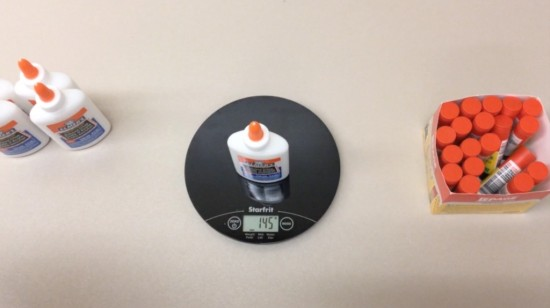The weight of one glue stick is 21 grams: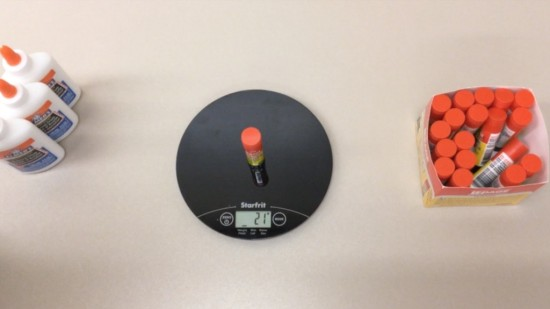At this point, your students should notice that their answers are close to these weights, but not exact.

Why might that be?

What extraneous variables could influence the results?

# Task 2: Write N’ Erase

## Act 1 – Introduce The Problem

Show students this video.

They will already know the question, so move on to Act 2…

## Act 2 – Reveal Some Information

Show students scene 1.

Alternatively, you can show students the following images:

### The weight of 13 pens and 5 packs of correction tape

The weight of 13 pens and 5 packs of correction tape is 166 grams: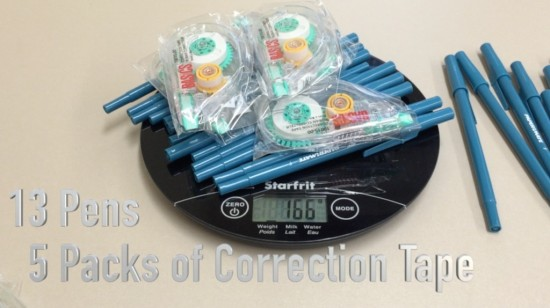### The weight of 6 pens and 3 packs of correction tape

The weight of 6 pens and 3 packs of correction tape is 88 grams: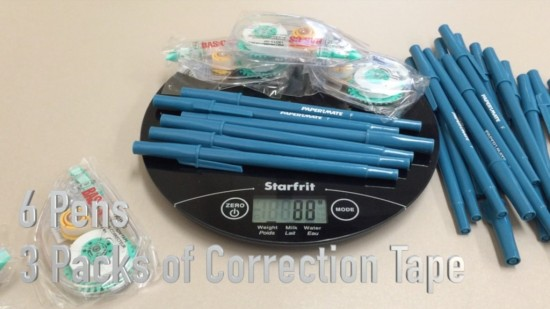Now, students can try to solve this system intuitively, by graphing or algebraically with substitution or elimination.

## Act 3 – Show The Solution

Show students the solution video.

Alternatively, you can show students these images:

The weight of one pen is 4 grams: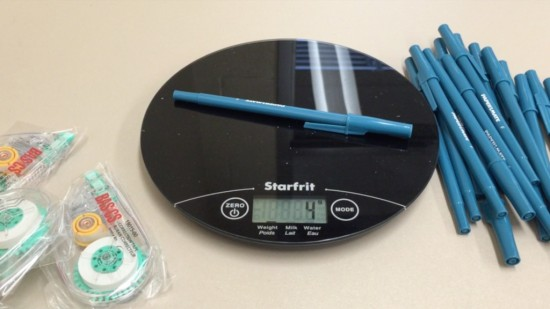The weight of one package of correction tape is 20 grams: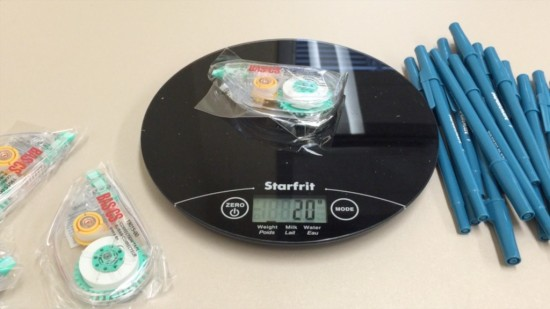Again, the actual weight may differ from what students found algebraically.

## Act 1 – Introduce The Problem

I find that sequel tasks like these often don’t require the “hook” as we’ve already engaged the learners to come towards the math. This task is simply additional practice prior to moving on to more traditional word problems and/or abstract problems. Thus, there is no act 1 video for this extension task.

## Act 2 – Reveal Some Information

Show students scene 1.

Alternatively, you can show students the following images:

The weight of 5 large notepads and 7 small notepads is 882 grams: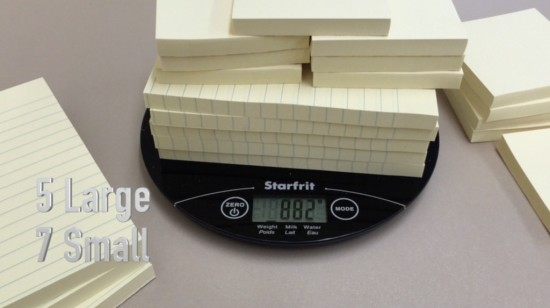The weight of 3 large notepads and 5 small notepads is 563 grams: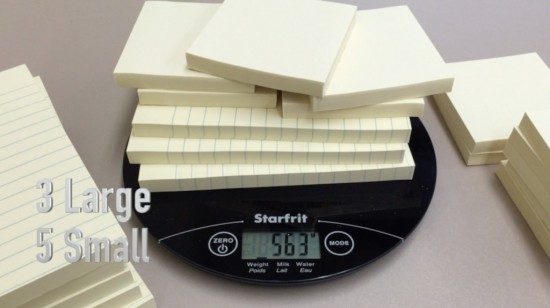Now, students can try to solve this system intuitively, by graphing or algebraically with substitution or elimination.

## Act 3 – Show The Solution

Show students the solution video.

Alternatively, you can show students these images:

The weight of one large notepad is 116 grams: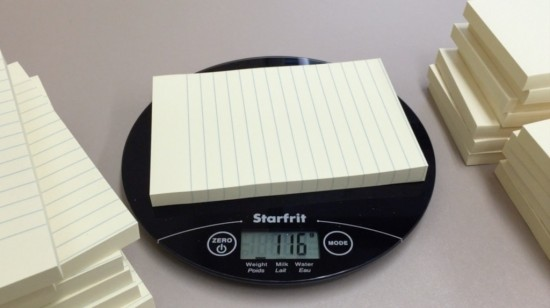The weight of one small notepad is 43 grams: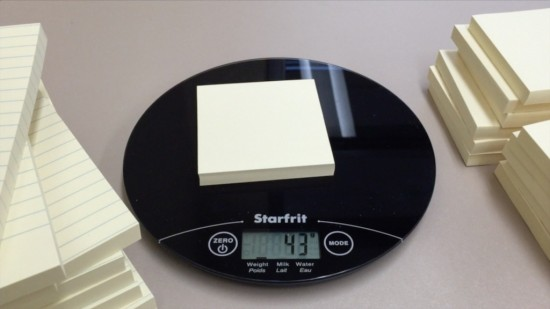Again, the actual weight may differ from what students found algebraically.

Try it out and let us know how it goes in the comments!

## New to Using 3 Act Math Tasks?

Download the 2-page printable 3 Act Math Tip Sheet to ensure that you have the best start to your journey using 3 Act math Tasks to spark curiosity and fuel sense making in your math classroom!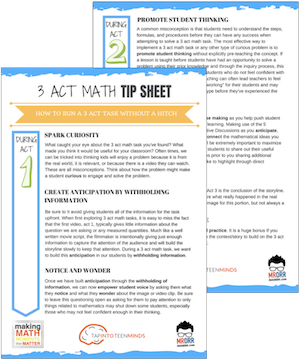## Share With Your Learning Community:I’m Kyle Pearce and I am a former high school math teacher. I’m now the K-12 Mathematics Consultant with the Greater Essex County District School Board, where I uncover creative ways to spark curiosity and fuel sense making in mathematics. Read more.

Access Other Real World Math Tasks

## Search More 3 Act Math Tasks

Spark Curiosity!